# (0,5,6,7,11) using: Implement the circuit defined by equation F(a,b,c,d) 1. 4-to-1 multiplexers and logic gates. 2....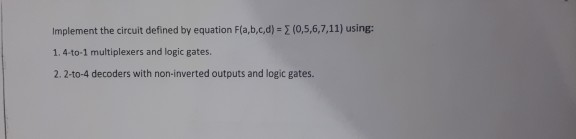(0,5,6,7,11) using: Implement the circuit defined by equation F(a,b,c,d) 1. 4-to-1 multiplexers and logic gates. 2. 2-to-4 decoders with non-inverted outputs and logic gates.The truth table for this: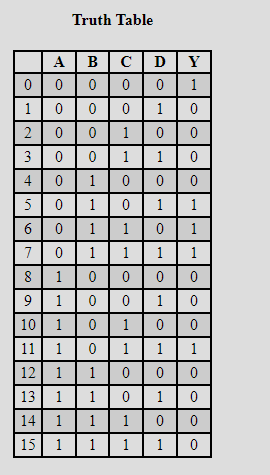K-- map of this: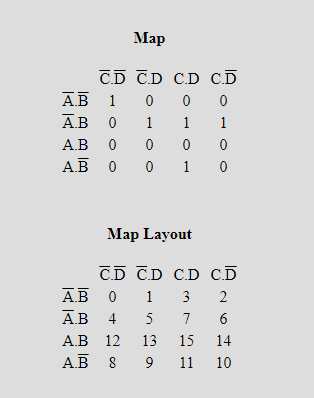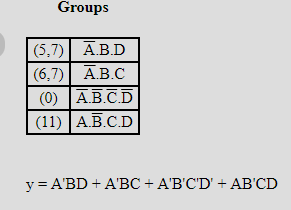Put S0 = A, And S1 = B

The truth table for S0 and  S1

 S0 S1 Y 0 0 D0 0 1 D1 1 0 D3 1 1 D2

Use MUX 4X1 :

D0 = C'.D'

D1 = C + D

D2 = 0 (because no pair form in this k- map)

D3 = C.D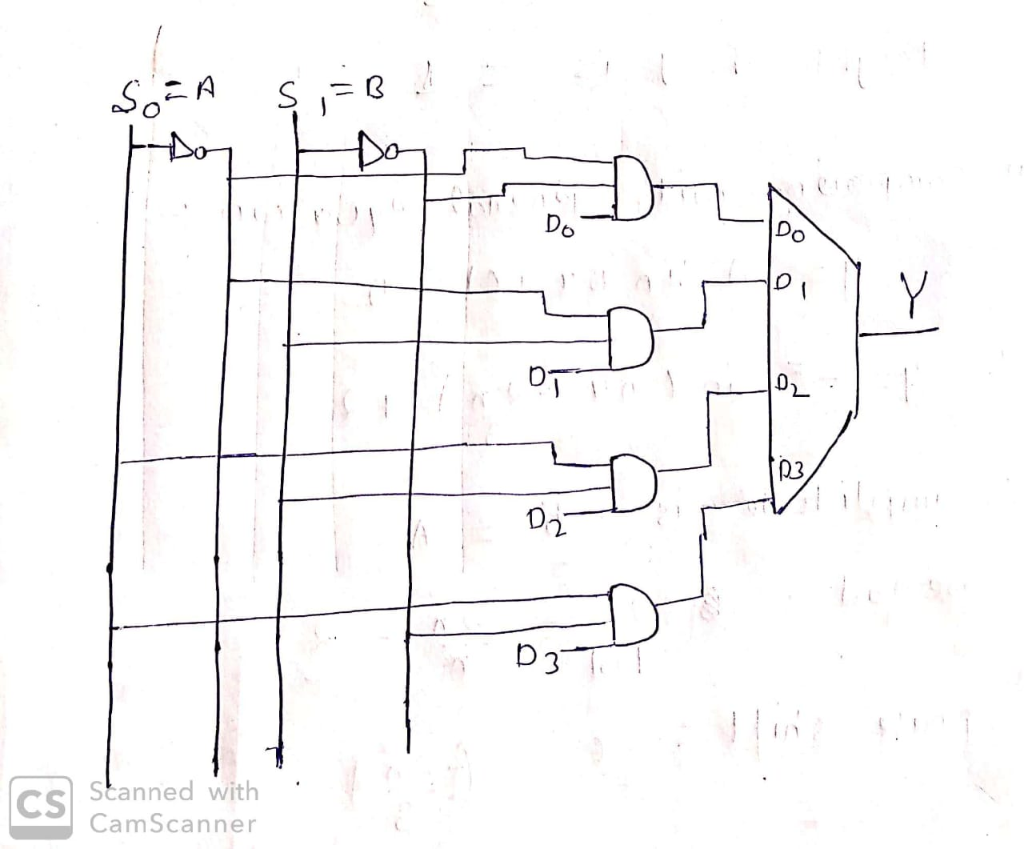For any doubt drop a comment.

Thank you!

##### Add Answer of: (0,5,6,7,11) using: Implement the circuit defined by equation F(a,b,c,d) 1. 4-to-1 multiplexers and logic gates. 2....
Similar Homework Help Questions
• ### Using building blocks such as binary adders, comparators, multiplexers, decoders, encoders, and arbiters as well as logic gates, design an 8x2 popularity circuit – a circuit that accepts eight two-bit...

Using building blocks such as binary adders, comparators, multiplexers, decoders, encoders, and arbiters as well as logic gates, design an 8x2 popularity circuit – a circuit that accepts eight two-bit numbers and outputs the number of times each of the four numbers appears on the input.

• ### Class 24 1. Given the shorthand POS expression F(a,b,c,d) П M (0,6,7,8) (b + c + d)(a + D+ ē): a....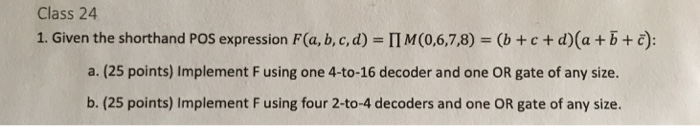Class 24 1. Given the shorthand POS expression F(a,b,c,d) П M (0,6,7,8) (b + c + d)(a + D+ ē): a. (25 points) Implement F using one 4-to-16 decoder and one OR gate of any size. b. (25 points) Implement F using four 2-40-4 decoders and one OR gate of any size. c. (25 points) Implement F using just two 8-to-3 encoders, NOT gates, and one AND gate of any size. Hint: given NOT gates and an AND gate to...

• ### multiplexers

Implement the function:R(a,b,c,d) = a'bc' + abcd + a'bd usinga) one 4-1 multiplexer and a few 2-1 multiplexersb) one 4-1 multiplexer with b and c as select inputs, and a few NAND2 logic gates at its input.c) a few 2-1 multiplexers as possible

• ### Digital Logic Design

For the following function F, whereA, B, C,D are all binary variables.IF (A = 0) THEN { IF(B = 0) THENF =D; ELSE F= 0; }ELSE{ IF (B = 0)THENF =C; ELSE F =1; }(a)Design a circuit that implements thefunction. You may use any combinational circuit component,including decoders, and multiplexers.(b) Repeat the same question without using anything other thanAND, OR, NOT. (Logic gates may have more than twoinputs.) The circuit should be as simpleas possible.

• ### all witworDFFs, FFI and FFo, two 4xI multiplexers, four 2-bit registers (Ro, RI, R2, and R3; all I with p arallel outputs) and no additional logic gates, design a circuit to support the following...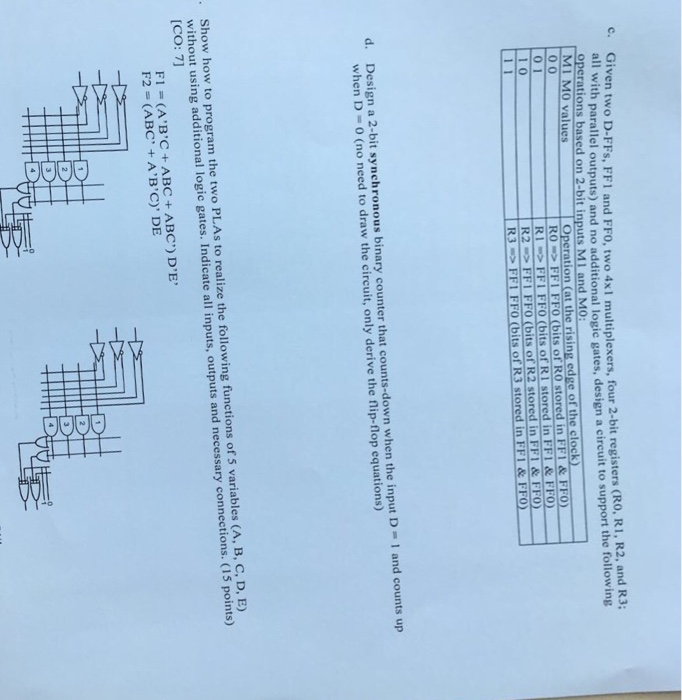all witworDFFs, FFI and FFo, two 4xI multiplexers, four 2-bit registers (Ro, RI, R2, and R3; all I with p arallel outputs) and no additional logic gates, design a circuit to support the following operations based on 2-bit inputs M1 and MO M1 MO values Operation (at the rising edge of the clock) RO FF1 FFO (bits of RO stored in FF1&FFO IFF1 FFO (bits of R1 stored in FF1&FFO R2 FF1 FFO (bits of R2 stored in FFI &FFO...

• ### Electric circuit logic (NOT, AND, OR gates), given inputs a,b,c and need to find !a !b !c by...

Electric circuit logic (NOT, AND, OR gates), given inputs a,b,cand need to find !a !b !c by using only 2 NOT gates and could useany amount of AND and OR gatesThus basicly, this is a questionwhich we are given 3 inputs (a, b, and c), and we will need tobuild a schematic circuit where we will get 3 outputs (!a, !b,!cwhich is the inverse of a,b,c) but we are only allowed to use 2 NOTgates in the whole schematic diagram,...

• ### Electric circuit logic (NOT, AND, OR gates), given inputs a,b,c and need to find !a !b !c by...

Electric circuit logic (NOT, AND, ORgates), given inputs a,b,c and need to find !a !b !c by using only2 NOT gates and could use any amount of AND and ORgatesThus basicly, this is a question which we are given 3 inputs(a, b, and c), and we will need to build a schematic circuit wherewe will get 3 outputs (!a, !b, !cwhich is the inverse of a,b,c)but we are only allowed to use 2 NOT gates in the whole schematicdiagram, and...

• ### Design a circuit for a 2:1 MUX using logic gates available

Design a circuit for a 2:1 MUX using logic gates available(AND,OR, and NOT)

• ### Implement the circuit using a suitable decoder and NAND gates

A combinational circuit is defined by the following 2 functions:F1(w, x, y) = ?m(0, 7)F2(w, x, y) = ?m(1,3,6)Implement the circuit using a suitable decoder and NAND gates.

• ### Logic ciruit using Nand Gates

Develop a logic circuit, using only NAND gates, to implement a circuit to meet the requirements of the truth table shown below.A B Y0 0 00 1 01 0 11 1 1

Need Online Homework Help?